# Entity list

Tags

Last time, you learned how to take an id from the URL, and show an entity with that id.

### Dish deets

For example, suppose we had a DB table called `dishes`, with data on fave dishes at the Mathieson's. It might look like this: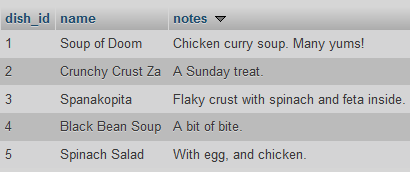There are three columns. The first one is an id number.

You could writ a program that would get a dish id from the URL, and show the dish's deets. Here's some output: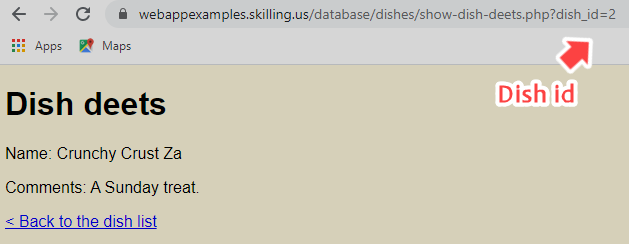You can try it.

Here's the code:

1. <?php
2. // To connect to a DB, need DB name, user name, and password.
3. \$dbName = 'dishes';
6. // Create DSN.
7. \$dsn = "mysql:host=localhost;dbname=\$dbName";
8. // Connect.
9. global \$dbConnection;
11. \$errorMessage = '';
12. // Get the id of the wombat the user wants to see.
13. \$id = getParamFromGet('dish_id');
14. \$errorMessage = checkDishId(\$id);
15. if (\$errorMessage == '') {
16.     // Prepare SQL.
17.     \$stmt = \$dbConnection->prepare('SELECT * FROM dishes WHERE dish_id = :id');
18.     // Run it.
19.     \$isQueryWorked = \$stmt->execute(['id' => \$id]);
20.     if (!\$isQueryWorked) {
21.         \$errorMessage = 'Sorry, something went wrong looking up the dish.';
22.     }
23.     else {
24.         \$row = \$stmt->fetch();
25.     }
26. }
27. ?><!DOCTYPE html>
28. ...
29. <h1>Dish deets</h1>
30. <?php
31. if (\$errorMessage != '') {
32.     print "<p class='error-message'>\$errorMessage</p>";
33. }
34. else {
35.     print "<p>Name: {\$row['name']}</p>";
37. }
38. ?>
39.
40. <?php
41.
42. ...
43.
44. /**
45.  * Check a dish id.
46.  * @param int \$dishId The id to check.
47.  * @return string Error message, MT if none.
48.  */
49. function checkDishId(\$dishId)
50. {
51.     \$errorMessage = '';
52.     // Missing?
53.     if (is_null(\$dishId)) {
54.         \$errorMessage = "Sorry, dish id is missing.<br>\n";
55.     }
56.     // Errors so far?
57.     if (\$errorMessage == '') {
58.         // Is numeric?
59.         if (!is_numeric(\$dishId)) {
60.             \$errorMessage = "Sorry, dish id must be a number, not '\$dishId'.<br>\n";
61.         }
62.     }
63.     // Errors so far?
64.     if (\$errorMessage == '') {
65.         // Is it big enough?
66.         if (\$dishId <= 0) {
67.             \$errorMessage = "Sorry, dish id of \$dishId is too small.<br>\n";
68.         }
69.     }
70.     // Return error message, if any.
71.     return \$errorMessage;
72. }

Nothing new here. Lines 1 to 10 connect to the DB. Lines 13 and 14 get an id from the URL, and check it. Lines 17 to 25 look up the dish with that id. Lines 31 to 37 output the dish's deets.

### Array of fields

Checkout this line:

• \$row = \$stmt->fetch();

PHP puts an array into `\$row`. An array is a collection of different values. In this case, each value is a field. Here's how it looks in memory, and how you access each field: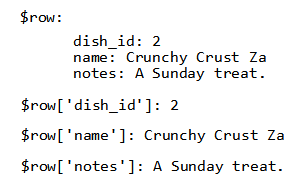The variable name doesn't matter. If we did this...

• \$dog = \$stmt->fetch();

...then we'd have: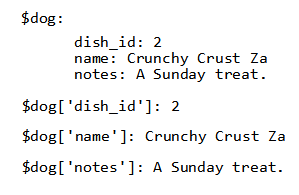OK, that's what we get when we fetch one row.

### Dish list

The URL of that page has an id at the end:

`http://webappexamples.skilling.us/database/dishes/show-dish-deets.php?dish_id=2`

We couldn't expect people to type id numbers in, when they wanted to see a dish. It would be better if they had a page with a list of dish names, and could click on the one they want: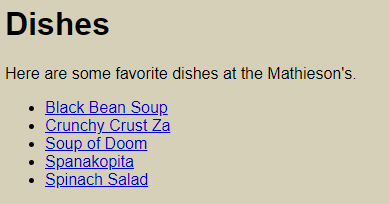That's what we'll do.

### The list HTML

You can see the list. Give it a trt.

Now check out the HTML. You'll see something like:

• <p>Here are some favorite dishes at the Mathieson's.</p>
• <ul>
• <li><a href='show-dish-deets.php?dish_id=4'>Black Bean Soup</a></li>
• <li><a href='show-dish-deets.php?dish_id=2'>Crunchy Crust Za</a></li>
• <li><a href='show-dish-deets.php?dish_id=1'>Soup of Doom</a></li>
• <li><a href='show-dish-deets.php?dish_id=3'>Spanakopita</a></li>
• </ul>

There's a link for each dish, matching the data in the DB:The order is different. The links are sorted by name. The DB data isn't.

The HTML for the link has the dish's id and name in it. If we set up the variables right, this PHP would make the link:

• \$link = "<a href='show-dish-deets.php?dish_id=\$id'> \$name </a>";

Code to make the list page would do this:

• Get all of the dish data
• For each dish:
•   \$id = dish id
•   \$name = dish name

### Gimme code!

Here it is:

1. <?php
2. // To connect to a DB, need DB name, user name, and password.
3. \$dbName = 'dishes';
6. // Create DSN.
7. \$dsn = "mysql:host=localhost;dbname=\$dbName";
8. // Connect.
9. global \$dbConnection;
11. \$errorMessage = '';
12. // Prepare SQL.
13. \$stmt = \$dbConnection->prepare('SELECT * FROM dishes ORDER BY name');
14. // Run it.
15. \$isQueryWorked = \$stmt->execute();
16. if (!\$isQueryWorked) {
17.     // Something broke.
18.     \$errorMessage = 'Sorry, something went wrong looking up the dishes.';
19. }
20. else {
21.     \$rows = \$stmt->fetchAll();
22. }
23. ?><!DOCTYPE html>
24. ...
25. <h1>Dishes</h1>
26. <?php
27. if (\$errorMessage != '') {
28.     print "<p class='error-message'>\$errorMessage</p>";
29. }
30. else {
31.     print "<p>Here are some favorite dishes at the Mathieson's.</p>\n";
32.     print "<ul>\n";
33.     // Loop across dishes.
34.     foreach (\$rows as \$row) {
35.         // Get data for one dish.
36.         \$id = \$row['dish_id'];
37.         \$name = \$row['name'];
38.         \$notes = \$row['notes'];
39.         // Make the link to the deets display page.
41.         // Output list item with link.
43.     }
44.     // End the list.
45.     print "</ul>\n";
46. }
47. ?>
48. </body>
49. </html>

Lines 1 to 10 connect to the DB. Line 13 sets up the query:

• \$stmt = \$dbConnection->prepare('SELECT * FROM dishes ORDER BY name');

There isn't any id here, since we want all the records in the table.

Then we run the SQL:

• \$isQueryWorked = \$stmt->execute();

The `execute()` doesn't have any parameters. It doesn't need any.

Next, we grab the data:

• \$rows = \$stmt->fetchAll();

Since we get more than one row back, we use `fetchAll()`, rather than `fetch()`. `fetchAll()` returns an array of rows. Each element is an array, the data for one row. Here's what it looks like in memory: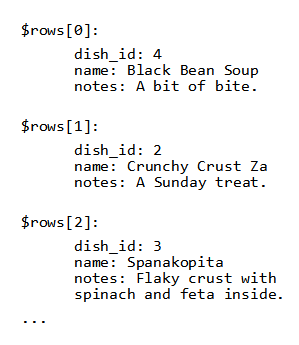​ The first item in the `\$rows` array is item `0`, the second one is item `1`, and so on. Why do they start at 0, and not 1? Because... don't worry about it. It has to do with memory pointers, the C language, and... stuff.

Here's the code that will run through `\$rows`:

• foreach (\$rows as \$row) {
•     // Get data for one dish.
•     \$id = \$row['dish_id'];
•     \$name = \$row['name'];
•     \$notes = \$row['notes'];
•     // Make the link to the deets display page.
PHP takes the first element in `\$rows`, puts it into the variable `\$row`, and runs the code inside the `foreach`. Then it takes the next element in `\$rows`, puts it into the variable `\$row`, and runs the code inside the `foreach`.
The goal is to make a page of links to deets about entities (wombats, meals, productions, whatevs). Make an SQL query that returns data about all of the entities you want to make links for. Use a `foreach` statement to loop over the records. For each one, generate a link to a page showing deets for that entity. The link will usually include an id.